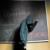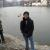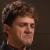## 代码没错又似乎错啦，请指点，c++

zhuceliyang 发布于 2012/11/07 19:33

为什么80%的码农都做不了架构师？->>>#include <iostream>
using namespace std;
class my
{
public:
my (int x,int y)
{
a=x;
b=y;
};
my()
{

};
void show(){
cout<<a<<endl<<b<<endl;
}
my(const my &p)
{
a=a*a;
b=b*b;
};
private:
int a,b;
};
int main(){
my m1(12,13),m2,m3=m1,m4(m1);//
m2=m1;
m1.show();
m2.show();
m3.show();
m4.show();
return 0;

}

12
13
12
13
687194768
687194768
687194768
687194768

0my(const my &p)
{
a=p.a*p.a;
b=p.b*p.b;

};好像是改成这样。。copy constructor不是这样用的吧

m2=m1使用的是赋值操作符，不会平方

0my(const my &p)

{
a=a*a;
b=b*b;
};

0#### 引用来自“优游幻世”的答案

my(const my &p)
{
a=p.a*p.a;
b=p.b*p.b;

};好像是改成这样。。copy constructor不是这样用的吧

m2=m1使用的是赋值操作符，不会平方

0#### 引用来自“xinzaibing”的答案

my(const my &p)

{
a=a*a;
b=b*b;
};